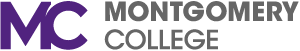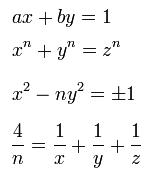# MathTalk: Diophantine Equations with Integer SolutionsCategory: Other

Published: 2011-11-17 00:00:01.000

Join us this Friday, November 18 at 2 p.m. in SW 010 for our next MathTalk.

Diophantine Equations with Integer Solutions
Professor Alex Bathula

Abstract: Integral solutions of equations with integral coefficients and with more than one unknown is a difficult problem in number theory. Famous mathematicians, Pythagoras, Diophantine, Fermat, Euler, Lagrange were concerned with this problem. Here we deal with a simple case of solving equations of first degree in two unknowns, i.e. solving ax+by=c in integers where a & b are non zero integers and c is an arbitrary integer and a and b are relatively prime. A useful theorem will be introduced along with some nice examples.

Related Media

1. diophantine.JPG